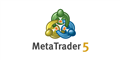# What could cause ea Initialization failed with code -1.

Please guys, this function is a vital part of my ea code but everytime I add it to my ea code, my ea fails to initialize though the code compiles with no errors. Thanks
```void GeetRightIndex(sNews &vNews[], sRight &conP[], sRight &conB[], int &fancy[])
{
string p_c,b_c;
int bpc=ArraySize(fancy);
p_c = SymbolInfoString(_Symbol, SYMBOL_CURRENCY_PROFIT);
b_c = SymbolInfoString(_Symbol, SYMBOL_CURRENCY_BASE);

for(int i=ArraySize(vNews)-1,j=0,w=0; i>=0; i--)
{
if(vNews[i].currency==p_c)
{
conP[j].rTime=(int)vNews[i].dTime;
conP[j].rIndex=i;
j++;
}
if(vNews[i].currency==b_c)
{
conB[w].rTime=(int)vNews[i].dTime;
conB[w].rIndex=i;
w++;
}
}
int b=ArraySize(conB), p=ArraySize(conP);

if((b==0 && p>0) || (p==0 && b>0))
{
fancy[bpc]=0;
bpc++;
}
else if(p>0 && b>0)
{
for (int x=fmin(p,b)-1; x>=0;x--)
{
if(fabs(conP[x].rTime-conB[x].rTime)>=3600)
{
if((vNews[(conB[x].rIndex)].currency=="USD") || (vNews[(conB[x].rIndex)].currency=="GBP") || (vNews[(conB[x].rIndex)].currency=="EUR"))
{
fancy[bpc]=conB[x].rIndex;
bpc++;
}
else
{
fancy[bpc]=conP[x].rIndex;
bpc++;
}
}
}
}
if(ArraySize(fancy)>=6)
{
int size=ArraySize(fancy)-1;
for(int i=4; i<size; i++)
{
fancy[i]= fancy[i+1];
}
ArrayResize(fancy, size);
}
if(ArraySize(conP)>=6)
{
int size=ArraySize(conP)-1;
for(int i=4; i<size; i++)
{
conP[i].rTime= conP[i+1].rTime;
conP[i].rIndex= conP[i+1].rIndex;
}
ArrayResize(conP, size);
}
if(ArraySize(conB)>=6)
{
int size=ArraySize(conB)-1;
for(int i=4; i<size; i++)
{
conP[i].rTime= conB[i+1].rTime;
conP[i].rIndex= conB[i+1].rIndex;
}
ArrayResize(conB,size);
}
}```

So... have you debugged your code?

ThankGod99 my ea fails to initialize though the code compiles with no errors.

Your posted code is irrelevant. OnInit is your initialization code, and it is returning non-zero.

William Roeder #:

Your posted code is irrelevant. OnInit is your initialization code, and it is returning non-zero.

I posted this because it seems to be the source of the error, I also see array out of range error in the experts tab, this part of the code is not even called in OnI
```void OnInit()
{
if(!MQLInfoInteger(MQL_TESTER) || !MQLInfoInteger(MQL_OPTIMIZATION))
{
DRAW_NEWS(NEWS_TABLE);

//TIME_CORRECTION=((int(TimeCurrent() - TimeGMT()) + 1800) / 3600);
TIME_CORRECTION=(-TimeGMTOffset());
}

double min_lot = SymbolInfoDouble(Symbol(), SYMBOL_VOLUME_MIN);
double lot_step = SymbolInfoDouble(Symbol(), SYMBOL_VOLUME_STEP);
Print("Minimum lot: ", DoubleToString(min_lot, 2), ", lot step: ", DoubleToString(lot_step, 2), ".");
if ((Lots < min_lot) && (!MM)) Alert("Lots should be not less than: ", DoubleToString(min_lot, 2), ".");

if (ShowTimer)
{
ObjectCreate(ChartID(), "NewsTraderTimer", OBJ_LABEL, 0, 0, 0);
}
else EventSetTimer(1);

// If UseATR = false, these values will be used. Otherwise, ATR values will be calculated later.
SL = StopLoss*10;
TP = TakeProfit*10;

}
```
nit. Here is my OnInit code.

ThankGod99:
Please guys, this function is a vital part of my ea code but everytime I add it to my ea code, my ea fails to initialize though the code compiles with no errors. Thanks
Carlos Moreno Gonzalez #:
So... have you debugged your code?
Nope, don't know how Sir.

ThankGod99 #:
Nope, don't know how Sir.

https://www.mql5.com/en/articles/2041 // Error Handling and Logging in MQL5
https://www.mql5.com/en/articles/272 // Tracing, Debugging and Structural Analysis of Source Code
https://www.mql5.com/en/articles/35 // scrol down to: "Launching and Debuggin"Code debugging - Developing programs - MetaEditor Help
MetaEditor has a built-in debugger allowing you to check a program execution step by step (by individual functions). Place breakpoints in the code...

ThankGod99 #:
Nope, don't know how Sir.

It's pretty easy. Set stop points so that your code stops there when executing, then you can go line by line, step over a function call, step out of it, etc. It's slow, of course, but really useful when you're stuck or wanna see if your code is doing what you're expecting. As a faster method and something more general, I suggest you put "Print" everywhere with a text that's telling you something, so that you see at a first glance if your code is entering an "if" or "for" or going here or there... that's definitely faster, but the debugger is something you're probably gonna need at some point. Take a look at the article posted in the previous reply - debugging is an essential part of developping. Good luck!

ThankGod99 this function is a vital part of my ea code but everytime I add it to my ea code, my ea fails to initialize though the code compiles with no errors. Thanks
ThankGod99 #: I posted this because it seems to be the source of the error, I also see array out of range error in the experts tab, this part of the code is not even called in OnI nit. Here is my OnInit code.

You said it's "fails to initialize" but posts initialize code that doesn't fail (void OnInit). Your initial statement is wrong.
How To Ask Questions The Smart Way. (2004)